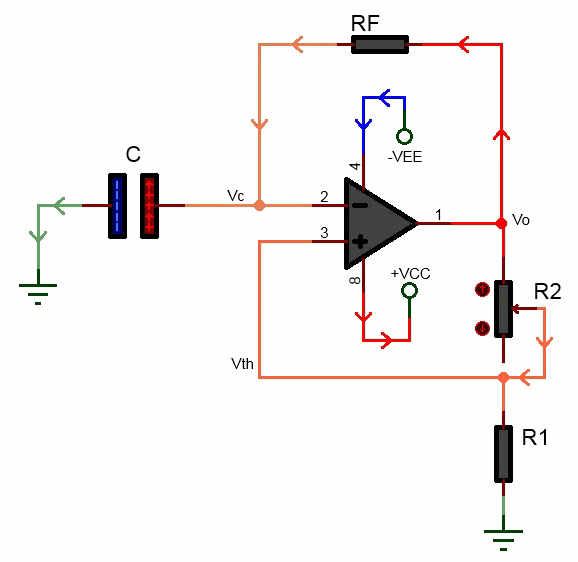# Op-Amp Astable Multivibrator Calculator

Calculate Frequency(fo):

V

Theoretical Results:The Op-Amp Astable Multivibrator Calculator is an online tool designed for electronics enthusiasts and professionals who work with Op-Amp Astable Multivibrators. This calculator allows users to easily calculate the feedback resistor value required to achieve a desired frequency, given the capacitor value and the feedback resistor values R1 and R2.

To use the calculator, the user simply enters the values of the capacitor, R1, R2, and the desired frequency, and the calculator then calculates the value of the feedback resistor (Rf) that is required to achieve the desired frequency. Additionally, the calculator displays the closest standard resistor values that can be used.

This calculator can be particularly useful for engineers, hobbyists, and students who are working on electronic projects that require an astable multivibrator. For example, it can be used to design oscillators, signal generators, and timing circuits. It can also be used to troubleshoot existing circuits that are not functioning properly, by allowing the user to quickly calculate the feedback resistor value needed to adjust the frequency of the circuit.

By making this calculator available online, users can easily access it from anywhere with an internet connection, and it can be used on various devices such as desktop computers, laptops, tablets, and smartphones. Additionally, since the calculator is web-based, users don't need to download any software or plugins to use it.

Overall, the Op-Amp Astable Multivibrator Calculator is a useful and convenient tool for anyone working with Op-Amp Astable Multivibrators. It simplifies the process of calculating the feedback resistor value needed for a particular frequency, saving users time and effort in their projects. It can also be helpful for students learning about Op-Amp Astable Multivibrators, as it provides a quick and easy way to verify their calculations.

Op-Amp Astable Multivibrator formulat or equation:

$$f_o = \frac{1}{2R_F C ln(\frac{-V_{sat}-V_{LT}}{+V_{sat}-V_{LT}})}$$  ---->(1)
$$V_{UT}=\frac{R_1 V_{sat}}{R_1+R_2}$$   ------->(2)
$$V_{LT}=\frac{-R_1 V_{sat}}{R_1+R_2}$$  ----------->(3)

Following are related multivibrator circuits one can alternative use.

- Monostable Multivibrator using Transistors
- 555 Timer as Astable Multivibrator